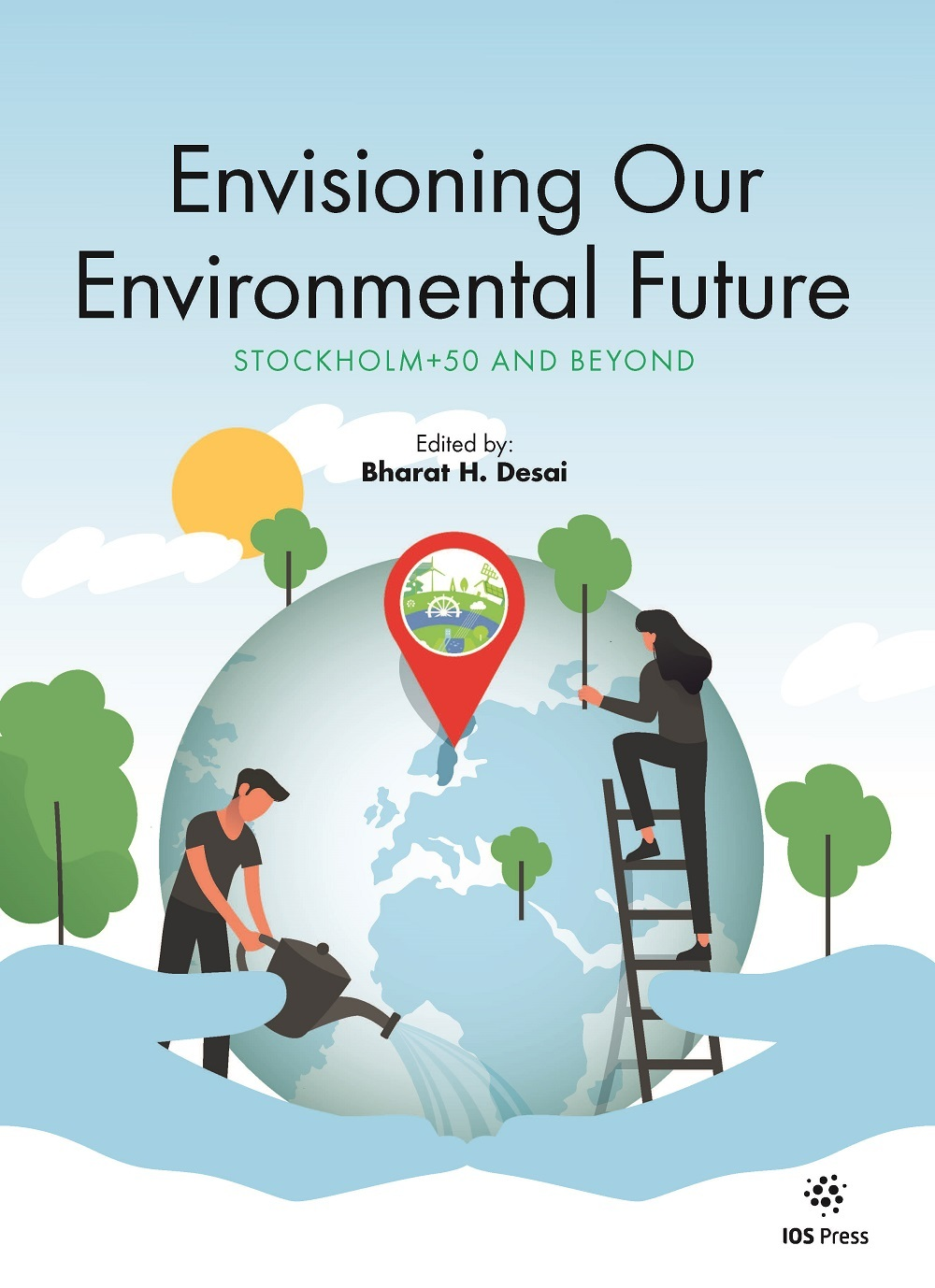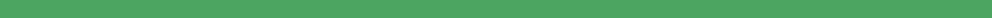Like   Tweet   in
 /* styles */ Please feel free forward to your colleagues and share the news of our new book from EPL

# ENVISIONING OUR ENVIRONMENTAL FUTURE

## New Book Out Now | Guest Editor: Bharat H. Desai, PhD

 table div table+table+table+table div table{width:100%;padding:0}table div table+table+table+table div table img{width:96.23%;padding:0;float:none}table div table+table+table+table div table td{width:100%;padding:0 1.88% 18px}/* styles */table.module-4{width:98.87%;padding:0}table div table+table+table+table+table div table{width:98.87%;float:none;margin-left:auto;margin-right:auto;padding:0}table div table+table+table+table+table div table a{border:0 none;text-decoration:none}table div table+table+table+table+table div table img{width:100%!important;border:0 none;text-decoration:none}table div table+table+table+table+table div table td{width:100%;padding:0}/* styles */

# Special Offer for our Readers

## Order Your Print Copy at a 20% Discount!*Discounted Print Book €108 / US\$133 / £98

As a special offer to our readers and the EPL community, you can now purchase the print book at a 20% discount.

Envisioning Our Environmental Future, with its 272 pages and 22 outstanding contributions, is currently available for a limited-time only at a discount price. Use the code below when ordering via our website before the end of December and you can get the print book for the discount price: €108 / US\$133 / £98.

Just place your order here before December 31, 2022 and enter the discount code listed below during the ordering process.

## DISCOUNT CODE: EPL20%ENVISION*

*Discount only applies to the print version; include the 14 digits of the code at checkout

 table div table+table+table+table+table+table+table+table div table{width:100%;padding:0}table div table+table+table+table+table+table+table+table div table img{width:96.23%;padding:0;float:none}table div table+table+table+table+table+table+table+table div table td{width:100%;padding:0 1.88% 18px}/* styles *//* styles */ About the Book Envisioning Our Environmental Future: Stockholm+50 and Beyond is a unique, limited-edition book. These are high-quality contributions published as a standalone volume by IOS Press. The content probes global environmental regulatory approaches and institutional effectiveness, and explores cutting-edge ideational solutions for our shared triple planetary crisis of climate change, biodiversity loss, and pollution. The 22 contributions are organized in three parts: Testing Times; Global Ideas; and Sectoral Ideas. About the Guest Editor Bharat H. Desai, PhD, is Professor of International Law and Jawaharlal Nehru Chair in International Environmental Law, Centre for International Legal Studies, School of International Studies, Jawaharlal Nehru University, New Delhi; and Editor-in-Chief of Environmental Policy and Law. Foreword Extract "This book recognizes the dire situation of our planet. The chapter authors analyze major issues relevant to the future and propose actions to conserve our planet for the future. Some chapters reflect on developments since Stockholm, others explore global issues, and still others examine challenges and proposals in specific sectors. Everyone can benefit from reading the volume." Edith Brown Weiss, Georgetown University, Washington DC, USA (source, in the freely available front matter PDF) View more about the book here & order your print copy with 20% discount here! This new book is a sequel to Our Earth Matters (Amsterdam: IOS Press, 2021)
 table div table+table+table+table+table+table+table+table+table+table div table td,table.module-9{width:100%;padding:0}table div table+table+table+table+table+table+table+table+table+table div table{width:100%;float:none;margin-left:auto;margin-right:auto;padding:0}table div table+table+table+table+table+table+table+table+table+table div table a{border:0 none;text-decoration:none}table div table+table+table+table+table+table+table+table+table+table div table img{width:100%!important;border:0 none;text-decoration:none}/* styles */
 table div table+table+table+table+table+table+table+table+table+table+table+table div table{width:100%;padding:0}table div table+table+table+table+table+table+table+table+table+table+table+table div table img{width:96.23%;padding:0;float:none}table div table+table+table+table+table+table+table+table+table+table+table+table div table td{width:100%;padding:0 1.88% 18px}/* styles */table.module-12{width:99.43%;padding:0}table div table+table+table+table+table+table+table+table+table+table+table+table+table div table{width:99.43%;float:none;margin-left:auto;margin-right:auto;padding:0}table div table+table+table+table+table+table+table+table+table+table+table+table+table div table a{border:0 none;text-decoration:none}table div table+table+table+table+table+table+table+table+table+table+table+table+table div table img{width:100%!important;border:0 none;text-decoration:none}table div table+table+table+table+table+table+table+table+table+table+table+table+table div table td{width:100%;padding:0}/* styles */

## View Related News Posts

Head to our website to read the latest news items. Did you know that we invite our journal community to submit posts (see here). Read the recent such post by Martin Wolf (Yale Center for Environmental Law and Policy) about the EPI via the link below.

Recent Blog Posts

 table div table+table+table+table+table+table+table+table+table+table+table+table+table+table+table div table,table.module-14{width:44.34%;float:left;padding:0}table div table+table+table+table+table+table+table+table+table+table+table+table+table+table+table div table a{border:0 none;text-decoration:none}table div table+table+table+table+table+table+table+table+table+table+table+table+table+table+table div table img{width:100%!important;border:0 none;text-decoration:none}table div table+table+table+table+table+table+table+table+table+table+table+table+table+table+table div table td{width:100%;padding:0 20px 20px 0}/* styles */ Watch the Recording of Recent EPL Webinar EPL's Editor-in-Chief Bharat H. Desai, PhD, led an expert panel discussion during the webinar covering the decision of the United Nations General Assembly to recognize the Human Right to a Clean, Healthy, and Sustainable Environment. View
 table div table+table+table+table+table+table+table+table+table+table+table+table+table+table+table+table div table,table.module-15{width:43.4%;float:left;padding:0}table div table+table+table+table+table+table+table+table+table+table+table+table+table+table+table+table div table a{border:0 none;text-decoration:none}table div table+table+table+table+table+table+table+table+table+table+table+table+table+table+table+table div table img{width:100%!important;border:0 none;text-decoration:none}table div table+table+table+table+table+table+table+table+table+table+table+table+table+table+table+table div table td{width:100%;padding:0 20px 20px 0}/* styles */ New Book – Order with Discount Following on from EPL's special section entitled Stockholm+50 and Beyond, all the contributions have now been collated and published in a unique and limited-edition book. EPL's Editor-in-Chief Bharat H. Desai, PhD, has curated the content to include many cutting-edge ideational reflections. View
 table div table+table+table+table+table+table+table+table+table+table+table+table+table+table+table+table+table div table,table.module-16{width:44.53%;float:left;padding:0}table div table+table+table+table+table+table+table+table+table+table+table+table+table+table+table+table+table div table a{border:0 none;text-decoration:none}table div table+table+table+table+table+table+table+table+table+table+table+table+table+table+table+table+table div table img{width:100%!important;border:0 none;text-decoration:none}table div table+table+table+table+table+table+table+table+table+table+table+table+table+table+table+table+table div table td{width:100%;padding:0 20px 20px 0}/* styles */ Repurposed UN Trusteeship Council This post is based on a proposal first published in the recent issue EPL and the new book Envisioning Our Environmental Future: Stockholm+50 and Beyond. This proposal holds significance in view of a flurry of initiatives taken by the United Nations Secretary-General (UNSG) António Guterres. View
 table div table+table+table+table+table+table+table+table+table+table+table+table+table+table+table+table+table+table div table,table.module-17{width:44.53%;float:left;padding:0}table div table+table+table+table+table+table+table+table+table+table+table+table+table+table+table+table+table+table div table a{border:0 none;text-decoration:none}table div table+table+table+table+table+table+table+table+table+table+table+table+table+table+table+table+table+table div table img{width:100%!important;border:0 none;text-decoration:none}table div table+table+table+table+table+table+table+table+table+table+table+table+table+table+table+table+table+table div table td{width:100%;padding:0 20px 20px 0}/* styles */ Environmental Performance Index (EPI) Discover how the EPI (produced by researchers at Yale and Columbia Universities) leverages cutting-edge scientific insights and the latest data to highlight the best environmental policy practices and move countries around the world towards a more sustainable future. View
 table div table+table+table+table+table+table+table+table+table+table+table+table+table+table+table+table+table+table+table+table div table td,table.module-19{width:100%;padding:0}table div table+table+table+table+table+table+table+table+table+table+table+table+table+table+table+table+table+table+table+table div table{width:100%;float:none;margin-left:auto;margin-right:auto;padding:0}table div table+table+table+table+table+table+table+table+table+table+table+table+table+table+table+table+table+table+table+table div table a{border:0 none;text-decoration:none}table div table+table+table+table+table+table+table+table+table+table+table+table+table+table+table+table+table+table+table+table div table img{width:100%!important;border:0 none;text-decoration:none}/* styles */
 table div table+table+table+table+table+table+table+table+table+table+table+table+table+table+table+table+table+table+table+table+table div table{width:100%;padding:0}table div table+table+table+table+table+table+table+table+table+table+table+table+table+table+table+table+table+table+table+table+table div table img{width:96.23%;padding:0;float:none}table div table+table+table+table+table+table+table+table+table+table+table+table+table+table+table+table+table+table+table+table+table div table td{width:100%;padding:0 1.88% 18px}/* styles */table.module-21{width:99.43%;padding:0}table div table+table+table+table+table+table+table+table+table+table+table+table+table+table+table+table+table+table+table+table+table+table div table{width:99.43%;float:none;margin-left:auto;margin-right:auto;padding:0}table div table+table+table+table+table+table+table+table+table+table+table+table+table+table+table+table+table+table+table+table+table+table div table a{border:0 none;text-decoration:none}table div table+table+table+table+table+table+table+table+table+table+table+table+table+table+table+table+table+table+table+table+table+table div table img{width:100%!important;border:0 none;text-decoration:none}table div table+table+table+table+table+table+table+table+table+table+table+table+table+table+table+table+table+table+table+table+table+table div table td{width:100%;padding:0}/* styles */

EPL publishes content that facilitates an understanding of environmental policy and environmental law issues. It is a global journal that seeks to publish cutting-edge scholarly works that have global significance. EPL provides a platform to facilitate an ideational understanding of international environmental policy, law, and institutional issues.

## Why submit to our EPL journal?

Benefits for contributing authors include:
• Rapid online publication (pre-press)
• Hassle free submission process via Editorial Manager (see here)
• Structured peer review thanks to the input of our Editorial Board
• Open access options – see IOS Press Open Library for details

 table div table+table+table+table+table+table+table+table+table+table+table+table+table+table+table+table+table+table+table+table+table+table+table+table+table div table{width:100%;padding:0}table div table+table+table+table+table+table+table+table+table+table+table+table+table+table+table+table+table+table+table+table+table+table+table+table+table div table img{width:96.23%;padding:0;float:none}table div table+table+table+table+table+table+table+table+table+table+table+table+table+table+table+table+table+table+table+table+table+table+table+table+table div table td{width:100%;padding:0 1.88% 18px}/* styles */table.module-25{width:99.43%;padding:0}table div table+table+table+table+table+table+table+table+table+table+table+table+table+table+table+table+table+table+table+table+table+table+table+table+table+table div table{width:99.43%;float:none;margin-left:auto;margin-right:auto;padding:0}table div table+table+table+table+table+table+table+table+table+table+table+table+table+table+table+table+table+table+table+table+table+table+table+table+table+table div table a{border:0 none;text-decoration:none}table div table+table+table+table+table+table+table+table+table+table+table+table+table+table+table+table+table+table+table+table+table+table+table+table+table+table div table img{width:100%!important;border:0 none;text-decoration:none}table div table+table+table+table+table+table+table+table+table+table+table+table+table+table+table+table+table+table+table+table+table+table+table+table+table+table div table td{width:100%;padding:0}/* styles */table div table+table+table+table+table+table+table+table+table+table+table+table+table+table+table+table+table+table+table+table+table+table+table+table+table+table+table+table+table+table+table div table{width:100%;padding:0}table div table+table+table+table+table+table+table+table+table+table+table+table+table+table+table+table+table+table+table+table+table+table+table+table+table+table+table+table+table+table+table div table img{width:96.23%;padding:0;float:none}table div table+table+table+table+table+table+table+table+table+table+table+table+table+table+table+table+table+table+table+table+table+table+table+table+table+table+table+table+table+table+table div table td{width:100%;padding:0 1.88% 18px}/* styles */